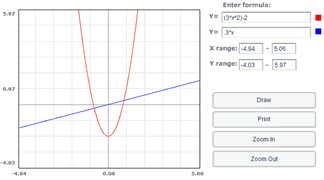# Helpful Online Calculators

This is your one-stop page for the various "solver" tools we have available. Consider bookmarking the page for quick access! Note that most of these tools are provided by MathWay, but there's no need to leave our site to get your answer. This is a GREAT tool if you're looking to check your work, or perhaps solve a tricky problem in an engineering class. But remember, if you're doing homework for your math class, your teacher will want to see your work completed thoroughly, not just an answer.

Equation Solver: Solve for x: $$x^2-8x+7=0$$

Equation GrapherDerivative Calculator:$$\frac{d}{dx} x^2-8x+C$$

Factoring Calculator: Factor $$x^2-3x+2=0$$

Integral Calculator: $$\int_{0}^{\pi} \sin x \, dx$$

Limit Calculator: $$\lim_{x \to +\infty} \frac{3x^2 +7x^3}{x^2 +5x^4}$$

Antiderivative Calculator

Summation Calculator

Matrix Calculator